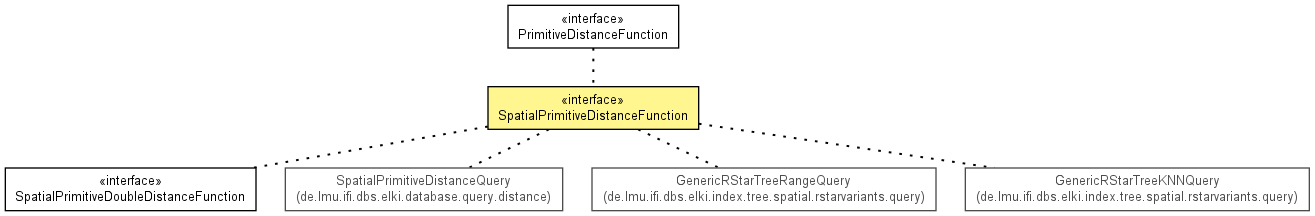de.lmu.ifi.dbs.elki.distance.distancefunction

## Interface SpatialPrimitiveDistanceFunction<V extends SpatialComparable,D extends Distance<D>>• ### Method Summary

Methods
Modifier and Type Method and Description
`<T extends V> SpatialDistanceQuery<T,D>` `instantiate(Relation<T> relation)`
Instantiate with a database to get the actual distance query.
`D` ```minDist(SpatialComparable mbr1, SpatialComparable mbr2)```
Computes the distance between the two given MBRs according to this distance function.
• ### Methods inherited from interface de.lmu.ifi.dbs.elki.distance.distancefunction.PrimitiveDistanceFunction

`distance, getInputTypeRestriction`
• ### Methods inherited from interface de.lmu.ifi.dbs.elki.distance.distancefunction.DistanceFunction

`getDistanceFactory, isMetric, isSymmetric`
• ### Method Detail

• #### minDist

```D minDist(SpatialComparable mbr1,
SpatialComparable mbr2)```
Computes the distance between the two given MBRs according to this distance function.
Parameters:
`mbr1` - the first MBR object
`mbr2` - the second MBR object
Returns:
the distance between the two given MBRs according to this distance function
• #### instantiate

`<T extends V> SpatialDistanceQuery<T,D> instantiate(Relation<T> relation)`
Description copied from interface: `DistanceFunction`
Instantiate with a database to get the actual distance query.
Specified by:
`instantiate` in interface `DistanceFunction<V extends SpatialComparable,D extends Distance<D>>`
Parameters:
`relation` - The representation to use
Returns:
Actual distance query.
 Release 0.5.0 (2012-07-02_1155)